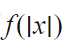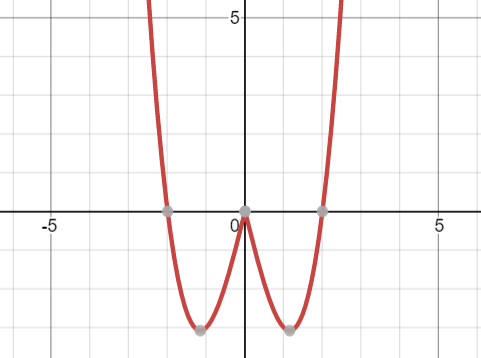# Mathematic Year 11 Ext Term 2 Exam # 2

Questions.

2.

1. Find the inverse function of y = -x2– 9 [3 marks]
2. Sketch the inverse function [3 marks]

3.

The polynomial 5x3 + 10x2 – 3 x + 2 has three roots α, β and γ

a) Find αβγ(α+β+γ) [1 marks]

b) Find α2 + β2 + γ2 [3 marks]

4.

Sketch y = x3 – 3x

5.

Sketch y = x4 – x2

6.

1. Find the inverse of this function, restrict the domain if required
2. Hence, or otherwise, sketch the inverse function

7.

1a) -1 is a root

b)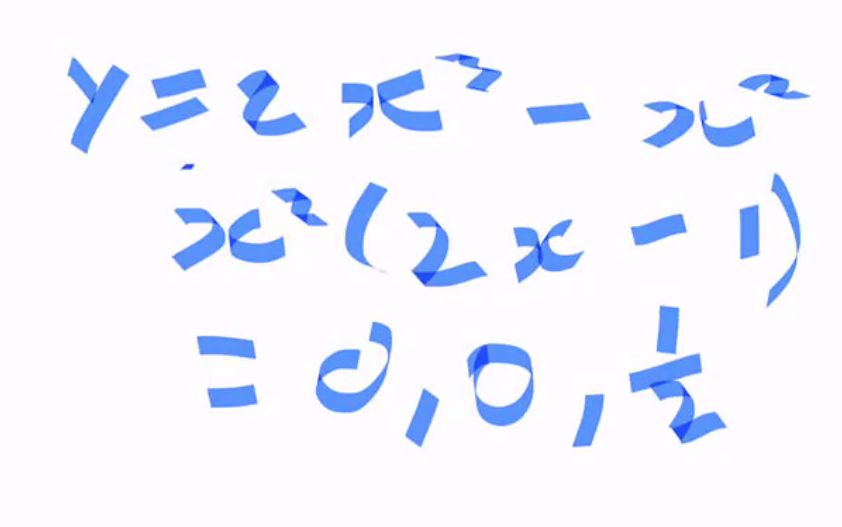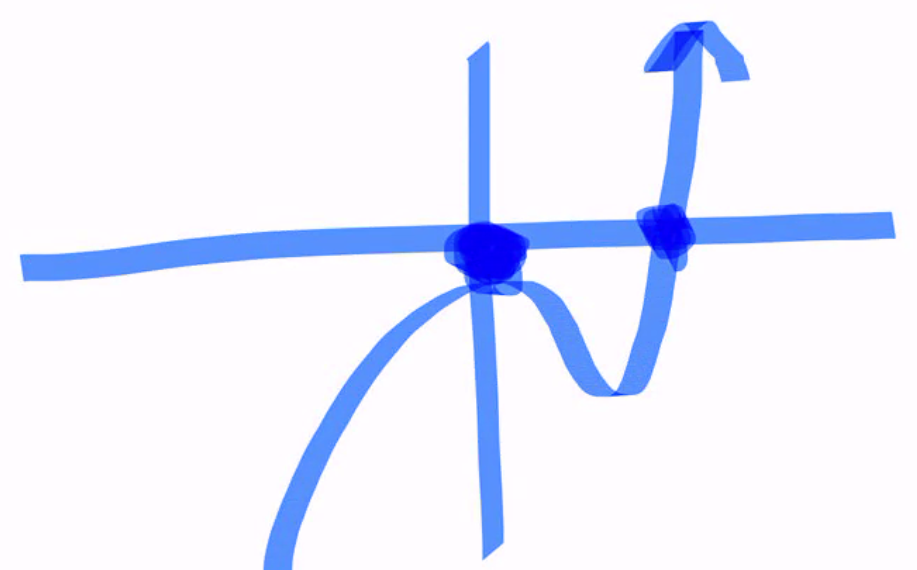The plus three then shifts everything up three places

Labels -1 is x-intercept and 3 is y-intercept

Question 2.

a)

b)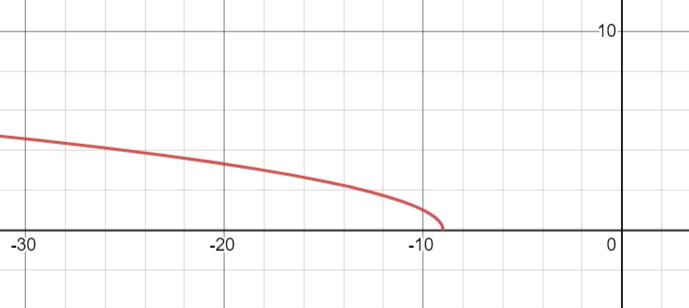Question 3.

1.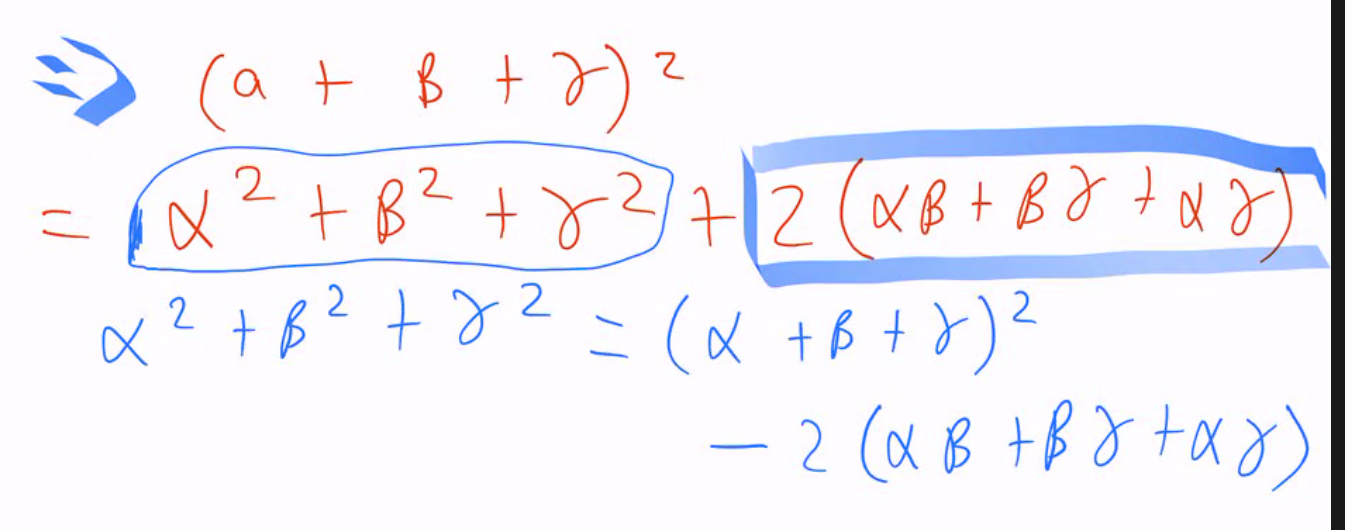26/5

Question 4.

Question 5.

Question 6.

a)

b)

Question 7.

1.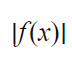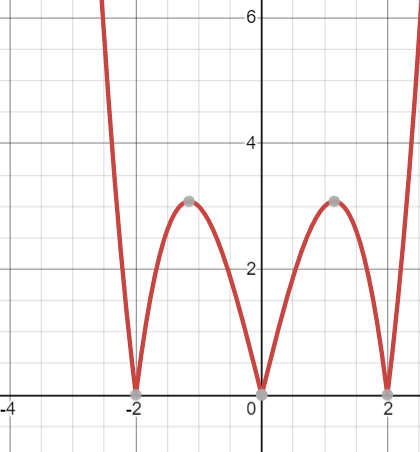2.